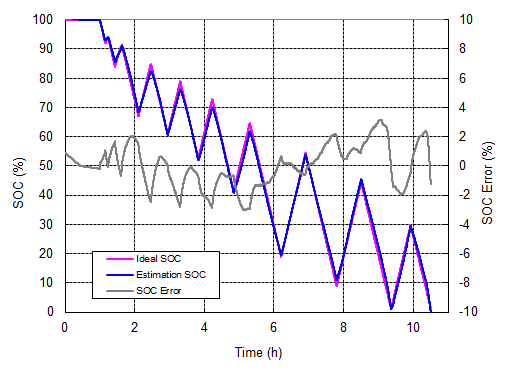1. Introduction of lithium-ion battery

1.1 State of Charge (State-Of-Charge; SOC)

State of charge can be defined as the state of available electrical energy in a battery, usually expressed as a percentage. Because the available electrical energy varies with charge and discharge current, temperature and aging phenomena, the definition of state of charge is also divided into two types: Absolute State-Of-Charge (ASOC) and Relative State of Charge (Relative State-of-Charge; ASOC) State-Of-Charge; RSOC). Usually the relative state of charge is in the range of 0% - 100%, while the battery is 100% when fully charged and 0% when fully discharged. The absolute state of charge is a reference value calculated from the designed fixed capacity value when the battery is manufactured. The absolute state of charge of a brand new fully charged battery is 100%; an aged battery, even fully charged, will not reach 100% under different charge and discharge conditions.

The graph below shows the relationship between voltage and battery capacity at different discharge rates. The higher the discharge rate, the lower the battery capacity. When the temperature is low, the battery capacity will also decrease.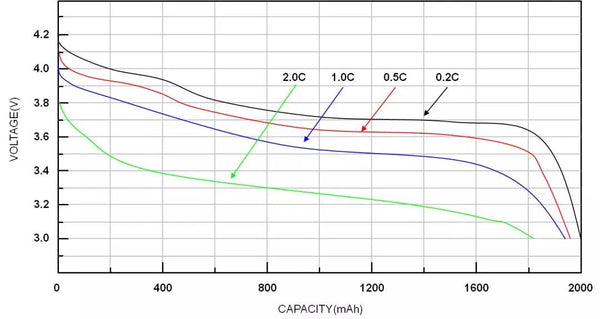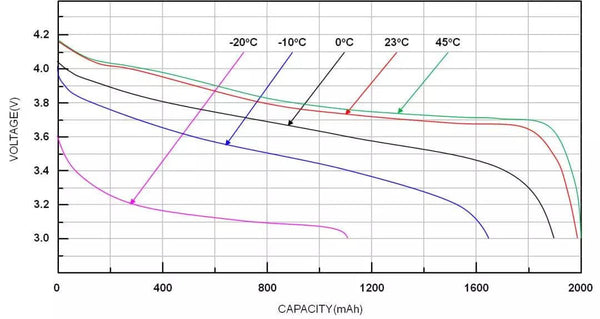1.2 Max Charging Voltage

Maximum charge voltage and battery chemistry are related to characteristics. The charging voltage of lithium batteries is usually 4.2V and 4.35V, and the voltage value will be different if the cathode and anode materials are different.

1.3 Fully Charged
When the difference between the battery voltage and the highest charging voltage is less than 100mV, and the charging current is reduced to C/10, the battery can be regarded as fully charged. Battery characteristics vary, as do full charge conditions.

The figure below shows a typical lithium battery charging characteristic curve. The battery is considered fully charged when the battery voltage is equal to the highest charging voltage and the charging current is reduced to C/10.Figure 2. Lithium battery charging characteristic curve

1.4 Mini Discharging Voltage
The minimum discharge voltage can be defined by the cut-off discharge voltage, which is usually the voltage at which the state of charge is 0%. This voltage value is not a fixed value, but varies with load, temperature, degree of aging, or others.

1.5 Fully Discharge
When the battery voltage is less than or equal to the minimum discharge voltage, it can be called full discharge.

1.6 Charge-discharge rate (C-Rate)
The charge-discharge rate is a representation of the charge-discharge current relative to the battery capacity. For example, after an hour of discharge at 1C, ideally, the battery will be fully discharged. Different charge and discharge rates will result in different usable capacities. Generally, the higher the charge-discharge rate, the smaller the usable capacity.

1.7 Cycle life
The number of cycles is the number of times a battery has undergone a complete charge and discharge, which can be estimated from the actual discharge capacity and the design capacity. Every time the accumulated discharge capacity is equal to the design capacity, the cycle number is once. Usually after 500 charge-discharge cycles, the capacity of a fully charged battery will drop by about 10% to 20%.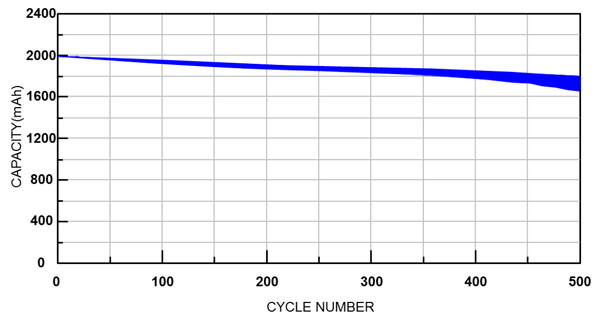1.8 Self-Discharge

The self-discharge of all batteries increases with temperature. Self-discharge is basically not a manufacturing flaw, but a characteristic of the battery itself. However, improper handling during the manufacturing process can also lead to an increase in self-discharge. Typically, the self-discharge rate doubles for every 10°C increase in battery temperature. The monthly self-discharge of lithium-ion batteries is about 1~2%, while the monthly self-discharge of various nickel-based batteries is 10~15%.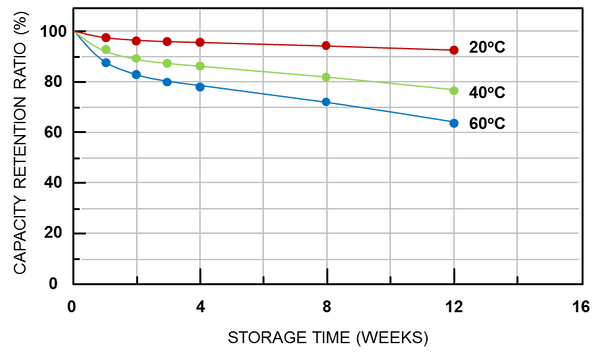2. Introduction to Battery Fuel Gauge
2.1 Introduction to the function of the fuel gauge

Battery management can be considered a part of power management. In battery management, the fuel gauge is responsible for estimating battery capacity. Its basic function is to monitor voltage, charge/discharge current and battery temperature, and to estimate battery state of charge (SOC) and battery full charge capacity (FCC). There are two typical methods for estimating the state of charge of a battery: the open circuit voltage method (OCV) and the coulometric method. Another method is the dynamic voltage algorithm designed by RICHTEK.

2.2 Open circuit voltage method
Using the open-circuit voltage method of the fuel gauge, its realization method is relatively easy, and can be obtained by looking up the table of the open-circuit voltage corresponding to the state of charge. The open circuit voltage is assumed to be the battery terminal voltage when the battery is resting for approximately more than 30 minutes.

Under different load, temperature, and battery aging conditions, the battery voltage curve will also be different. Therefore, a fixed open-circuit voltmeter cannot fully represent the state of charge; the state of charge cannot be estimated by looking up the table alone. In other words, if the state of charge is estimated only by looking up the table, the error will be large.

Figure 5. Battery voltage under charging and discharging conditions

As can be seen from the figure below, the state of charge varies greatly under different loads during discharge. So basically, the open circuit voltage method is only suitable for systems with low requirements on the accuracy of the state of charge, such as lead-acid batteries or uninterruptible power supplies in automobiles.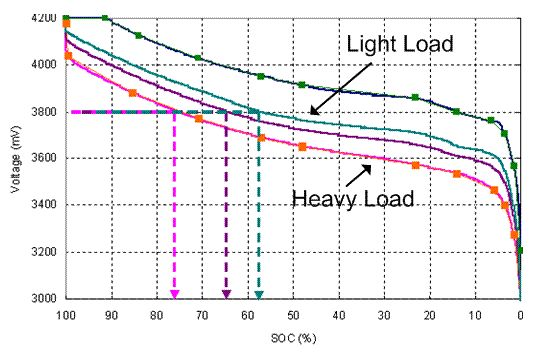2.3 Coulomb measurement
The principle of operation of the coulometric method is the connection of a sense resistor in the charge/discharge path of the battery. The ADC measures the voltage across the sense resistor and converts it to the current value of the battery being charged or discharged. A real-time counter (RTC) provides integration of this current value over time to know how many coulombs are flowing.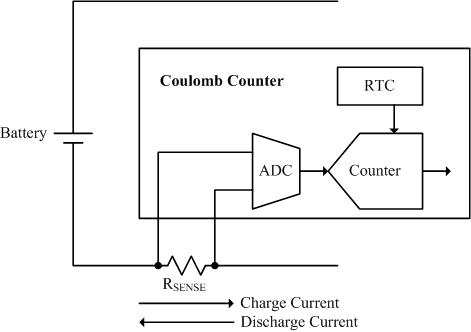Figure 7. Basic working method of Coulomb measurement
Coulomb metering can accurately calculate the real-time state of charge during charging or discharging. With charge coulomb counter and discharge coulomb counter, it can calculate remaining capacity (RM) and full charge capacity (FCC). Remaining capacity (RM) and full charge capacity (FCC) can also be used to calculate the state of charge, i.e. (SOC = RM / FCC). In addition, it can estimate the remaining time, such as power exhaustion (TTE) and power full (TTF).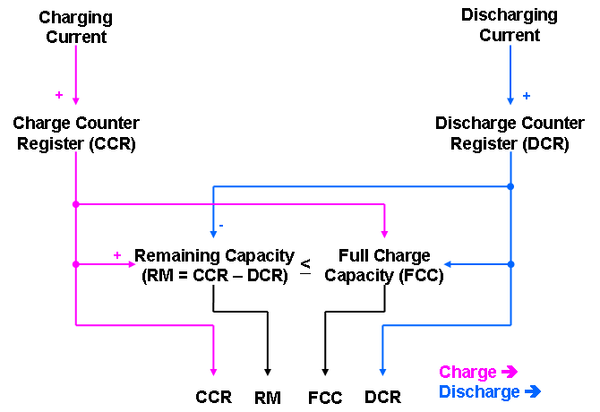Figure 8. Calculation formula of Coulomb measurement method
There are two main factors that contribute to the bias in the accuracy of coulomb measurement. The first is the accumulation of offset errors in current sensing and ADC measurements. Although this measurement error is relatively small with current technology, if there is no good way to eliminate it, the error will increase with time. The figure below shows that in practical applications, if there is no correction in the time duration, the accumulated error is unlimited.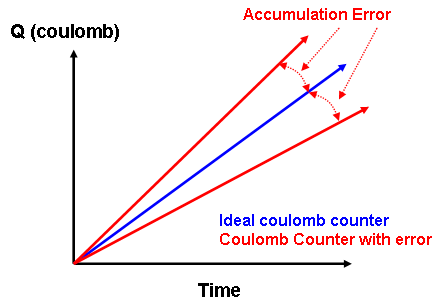Figure 9. Cumulative error of Coulomb measurement
To eliminate accumulated errors, there are three possible usable time points in normal battery operation: End of Charge (EOC), End of Discharge (EOD) and Rest (Relax). The end-of-charge condition is reached to indicate that the battery is fully charged and the state of charge (SOC) should be 100%. The end-of-discharge condition means that the battery is fully discharged and the state of charge (SOC) should be 0%; it can be an absolute voltage value or change with load. When a resting state is reached, the battery is neither charged nor discharged, and remains in this state for a long time. If the user wants to use the rest state of the battery to correct the error of the coulomb measurement method, it must be equipped with an open-circuit voltmeter at this time. The figure below shows that the state of charge error can be corrected for the above states.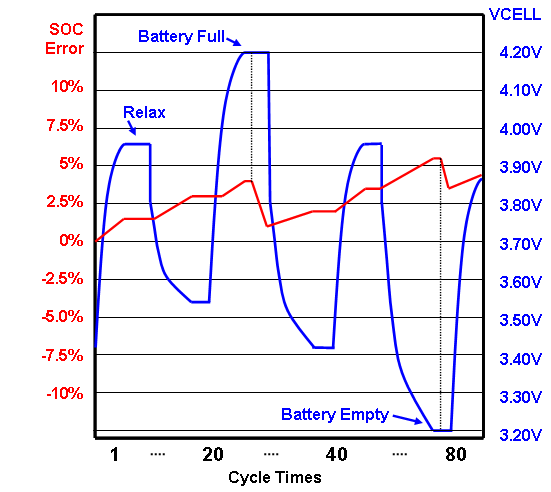Figure 10. Conditions for Eliminating the Cumulative Error of Coulomb Measurement

The second major contributor to the bias in coulometric accuracy is full charge capacity (FCC) error, which is the difference between the value of the battery's design capacity and the battery's true full charge capacity. Full charge capacity (FCC) will be affected by temperature, aging, load and other factors. Therefore, the relearning and compensation method of the full charge capacity is very important for the coulometric method. The figure below shows the trend of state of charge error when the full charge capacity is overestimated and underestimated.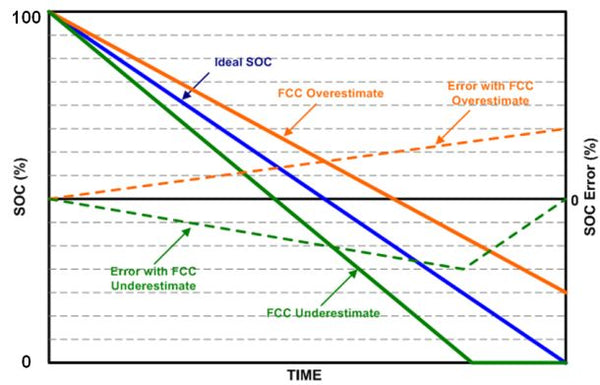Figure 11. Performance of Dynamic Voltage Algorithm Fuel Gauge and Gain Optimization
The following is the performance of the state of charge of the dynamic voltage algorithm under different discharge rate conditions. As can be seen from the figure, its state of charge accuracy is good. Regardless of the discharge conditions of C/2, C/4, C/7 and C/10, the overall state of charge error of this method is less than 3%.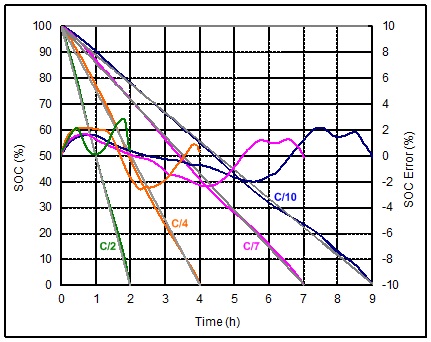Figure 12. The performance of the state of charge of the dynamic voltage algorithm under different discharge rates

The figure below shows the behavior of the state of charge when the battery is short-charged and short-discharged. The state-of-charge error is still small, with a maximum error of only 3%.